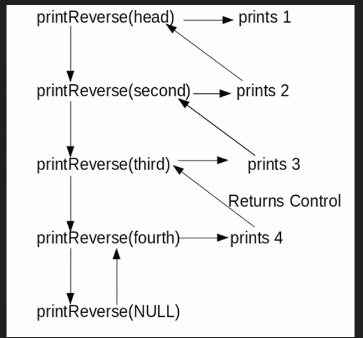GeeksforGeeks App
Open AppBrowser
Continue

# C Program For Printing Reverse Of A Linked List Without Actually Reversing

Given a linked list, print reverse of it using a recursive function. For example, if the given linked list is 1->2->3->4, then output should be 4->3->2->1.
Note that the question is only about printing the reverse. To reverse the list itself see this
Difficulty Level: RookieAlgorithm:

```printReverse(head)
1. call print reverse for head->next

Implementation:

## C

 `// C program to print reverse``// of a linked list``#include``#include`` ` `// Link list node``struct` `Node``{``    ``int` `data;``    ``struct` `Node* next;``};`` ` `// Function to reverse the linked list``void` `printReverse(``struct` `Node* head)``{``    ``// Base case ``    ``if` `(head == NULL)``       ``return``;` `    ``// Print the list after head node``    ``printReverse(head->next);` `    ``// After everything else is printed,``    ``// print head``    ``printf``(``"%d  "``, head->data);``}`` ` `// UTILITY FUNCTIONS``/* Push a node to linked list.``   ``Note that this function``   ``changes the head */``void` `push(``struct` `Node** head_ref,``          ``char` `new_data)``{``    ``// Allocate node``    ``struct` `Node* new_node =``           ``(``struct` `Node*) ``malloc``(``sizeof``(``struct` `Node));`` ` `    ``// Put in the data ``    ``new_node->data  = new_data;`` ` `    ``// Link the old list of the``    ``// new node``    ``new_node->next = (*head_ref);  `` ` `    ``// Move the head to point to the``    ``// new node``    ``(*head_ref)    = new_node;``}`` ` `// Driver code``int` `main()``{``    ``// Create linked list 1->2->3->4``    ``struct` `Node* head = NULL;   ``    ``push(&head, 4);``    ``push(&head, 3);``    ``push(&head, 2);``    ``push(&head, 1);``  ` `    ``printReverse(head);``    ``return` `0;``}`

Output:

`4 3 2 1`

Time Complexity: O(n)

Space Complexity: O(n) for call stack since using recursion

Please refer complete article on Print reverse of a Linked List without actually reversing for more details!

My Personal Notes arrow_drop_up